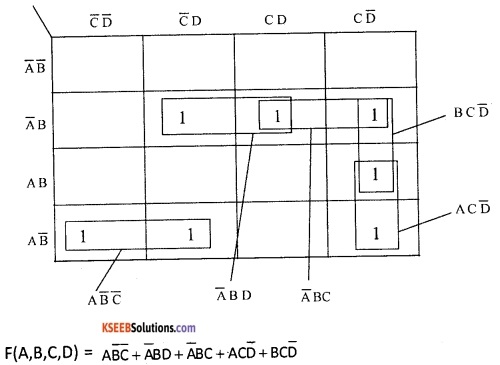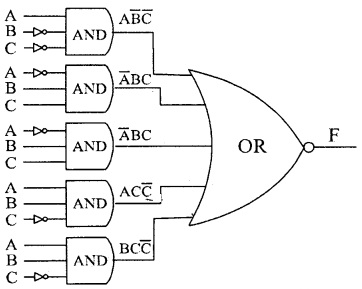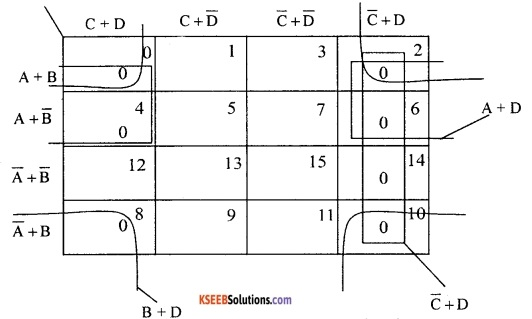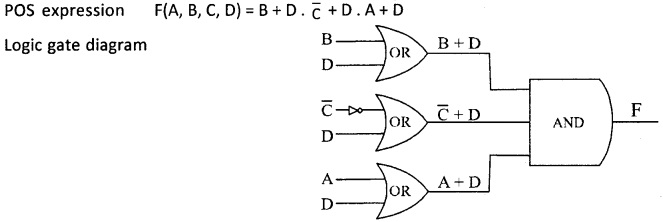# 2nd PUC Computer Science Question Bank Chapter 2 Boolean Algebra

## Karnataka 2nd PUC Computer Science Question Bank Chapter 2 Boolean Algebra

### 2nd PUC Computer Science Boolean Algebra One Mark Questions and Answers

Question 1.
What is another name of Boolean algebra?
Another name of Boolean algebra is ‘Switching Algebra’.

Question 2.
What do you understand by the term truth value?
The result TRUE or FALSE of logical statement are called truth values.

Question 3.
What do you understand by the term truth function?
The sentences which can be determined to be true or false are called truth function.

Question 4.
What do you meant by binary-valued variables?
The variables that can store TRUE (1) or FALSE (0) truth values are called binary-valued variables.Question 5.
What do you understand by logic function?
The logic function is a compound statement that consists of a logic statement with logical operators like AND, OR and NOT.

Question 6.
Give examples for logic function.
The examples for logic functions are
X NOT Y OR Z
Y AND X OR Z

Question 7.
What is meant by tautology and fallacy?
If the result of any logical statement or expression is always TRUE is called Tautology and the result of any logical statement is always FALSE is called fallacy.

Question8.
Prove the 1+Y is a tautology and 0.Y is a fallacy.

 tautology fallacy If Y=0, then 1 + 0=1 If Y = 1, then 0.1 = 0 If Y = 1, then 1+1 = 1 If Y = 0, then 0.0=0

Question 9.
Name the three logical operators.
The three logical operators are AND, OR and NOT.

Question 10.
What is a truth table? What is its significance?
Truth tables are a means of representing the results of a logic function using a table. They are constructed by defining all possible combinations of the inputs to a function and then calculating the output for each combination in turn.

Question 11.
What is NOT operator?
The “NOT” is simply the opposite or complement of its original value.

Question 12.
Write Venn diagram for NOT operator.
NOT – The first term but not the second is present.
fruit NOT apples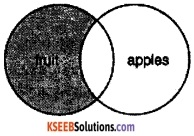Question 13.
Write the truth table for NOT operation.
The truth table for NOT operation.

 X NOT X 0 1 1 0

Question 14.
What is OR operator?
The OR is a disjunction operator and denotes logical addition.

Question 15.
Write Venn diagram for OR operator?
OR – Any one of the terms are present (more than one term may be present.
fruit OR vegetables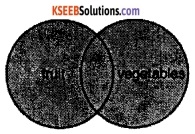fruit OR vegetables OR cereal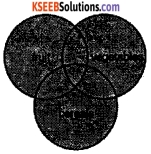Question 16.
Write the truth table for OR operator.

 X Y OUTPUT X + Y 0 0 0 0 1 1 1 0 1 1 1 1

Question 17.
What is AND operator?
The Logical AND operator is a conjunction operator and denotes logical multiplication.

Question 18.
Write Venn diagram for AND operator.
rivers AND salinity.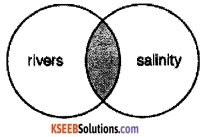Question 19.
Write the truth table for AND operation.

 X Y OUTPUT X.Y 0 0 0 0 1 0 1 0 0 1 1 1

Question 20.
State idempotent law.
When a variable combines with itself using OR or AND operator and produce the same variable as output is called idempotent law.
For example, X + X = X, X.X=X

Question 21.
Prove idempotent law using truth table.
Idempotent law using truth table

 X X OUTPUT X. X X + X 0 0 0 0 1 1 1 1

Question 22.
Draw logic diagram to represent idempotent law.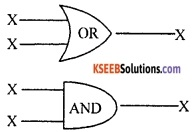Question 23.
State Involution law.
This law states that the double complement of a variable gives the same variable.

Question 24.
Prove Involution law using truth table.

 Y Y OUTPUT !(!Y) 0 1 0 1 O 1

Question 25.
Draw logic diagram to represent Involution law.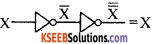Question 26.
State Complementarity law.
The complementarity law states that a term ANDed with its complement equals 0, and a term ORed with its complement equals 1 (AA’ = 0, A+A’ = 1).Question 27.
Prove Complementarity law using truth table.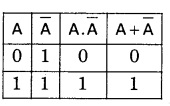Question 28.
Draw logic diagram to represent Complementarity law.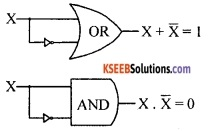Question 29.
State Commutative law.
The commutative law states that the order in which terms are written does not affect their value. For example, (AB = BA, A+B = B+A).

Question 30.
Prove Communicative law using truth table.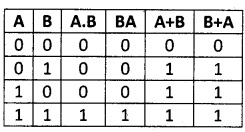Question 31.
Draw logic diagram to represent Commutative law.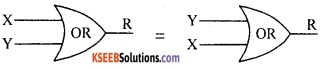Question 32.
State Associative law.
It is a simple equality statement i.e., A(BC) = ABC or A+(B+C) = A+B+C.

Question 33.
Prove Associative law using truth table.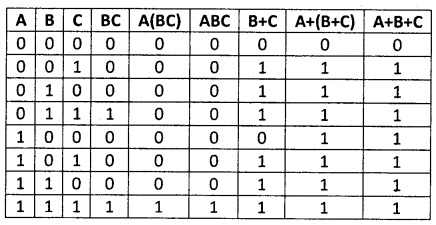Question 34.
Draw logic diagram to represent Associative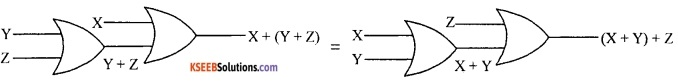Question 35.
State Distributive law.
1. a term (A) ANDed with an parenthetical expression (B+C) equals that term ANDed with each term within the parenthesis: A . (B+C) = AB+AC;

2. a term (A) ORed with a parenthetical expression (B . C) equals that term ORed with each term within the parenthesis: A+(BC) = (A+B) . (A+C).

Question 36.
Prove Distributive law using truth table.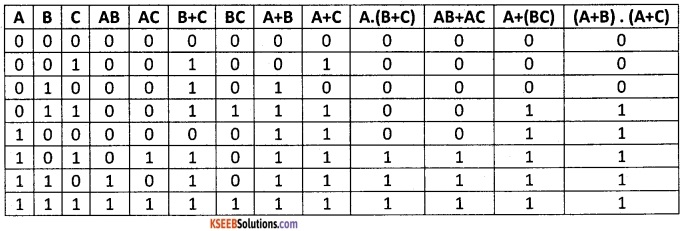Question 37.
Draw logic diagram to represent Distributive law.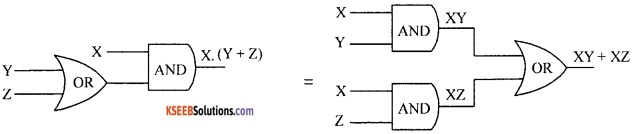Question 38.
Prove that X+XY = X (Absorption law)

 X Y X.Y X+XY 0 0 0 0 0 1 0 0 1 0 0 1 1 1 1 1

Question 39.
Prove that X(X+Y) = X (Absorption law).

 X Y X + Y X(X+Y) 0 0 0 0 0 1 1 0 1 0 1 1 1 1 1 1

Question 40.
Draw logic diagram to represent Absorption law.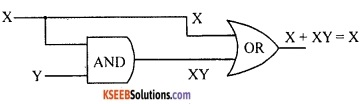Question 41.
Prove that XY + X$$\hat{\mathbf{Y}}$$ =X.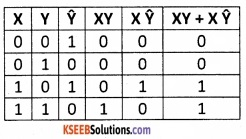Question 42.
Prove that (X+Y) (X+ $$\hat{\mathbf{Y}}$$) = X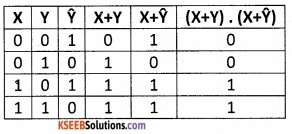Question 43.
Prove that A + $$\hat{\mathbf{A}}$$B = A + B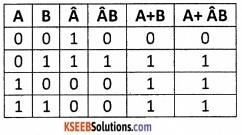Question 44.
What is minterm?
A minterm is a special product of literals, in which each input variable appears exactly once.

Question 45.
Find the minterm of AB + C.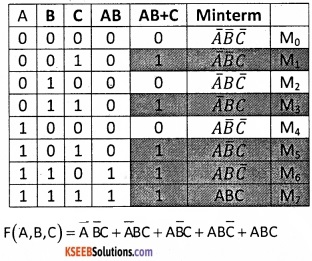Question 46.
What is a maxterm?
A maxterm is a sum of literals, in which each input variable appears exactly once.

Question 47.
Find the maxterm for X + $$\hat{\mathbf{Y}}$$ + Z.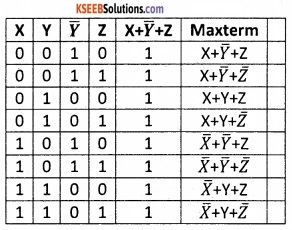Question 48.
What is the canonical form of Boolean expression?
Boolean expression expressed as sum of minterms or product of max terms are called canonical form.Question 49.
Give an example for a Boolean expression in the sum of minterms form.
The example for boolean expression is the sum of minterms form is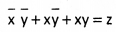Question 50.
Give an example for a Boolean expression in the product of max terms form.
The example for boolean expression in the product of max terms form is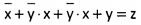### 2nd PUC Computer Science Boolean Algebra Two Marks Questions and Answers

Question 1.
Prove algebraically that (X+Y) (X+Z) = X + YZ
LHS: = (X+Y) (X+Z)
= XX + XZ + XY + YZ
= X + XZ + XY + YZ
= X + (1 + Z +Y) YZ
= X + YZ
= RHS

Question 2.
Prove algebraically that X+xY = X + Y
LHS : = X + $$\bar{x}$$ . X + Y
= 1 . X + Y
= X + Y

Question 3.
Use duality theorem to derive another Boolean relation from: A + $$\bar{A}$$ B = A+B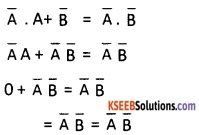Question 4.
What would be complement of the following: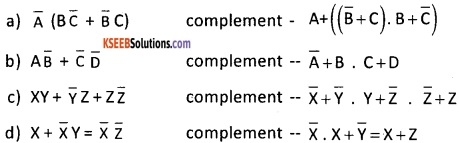Question 5.
What are the fundamental products for each of the input words?
ABCD = 0010, ABCD =0110, ABCD = 1110. Write SOP expression.
The SOP expression for ABCD = 0010
$$\bar{A}$$$$\bar{B}$$ C$$\bar{D}$$ = m2
The SOP expression for ABCD =0110,
$$\bar{A}$$ BC$$\bar{D}$$ = m6
The SOP expression for ABCD = 1110
ABC$$\bar{D}$$ = M14Question 6.
A truth table has output 1 for each of these input;
ABCD = 0011, ABCD =0101, ABCD = 10000, what are the fundamental products and write minterm expression.
Fundamental product of
ABCD = 0011 is $$\bar{A}$$ $$\bar{B}$$ C D = m3
ABCD = 0101 $$\bar{A}$$ B $$\bar{C}$$ D = m5
ABCD = 1000 is A $$\bar{B}$$ $$\bar{C}$$ $$\bar{D}$$ = m8
minterm expression is
f(A,B,C,D) = Σ(3, 5, 8)
or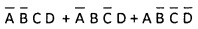Question 7.
Construct a Boolean function of three variables X, Y and Z that has an output 1 when exactly two of X, Y and Z are having values 0, and an output 0 in all other cases.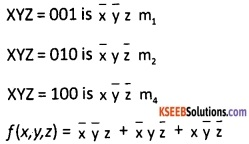Question 8.
Construct a truth table for three variables A, B and C that will have an output 1 when XYZ = 100, XYZ = 101, XYZ = 110 and XYZ = 111. Write the Boolean expression for logic network in the SOP form.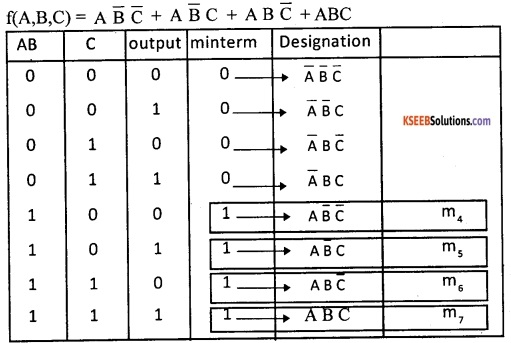Question 9.
Convert the following expressions to canonical Product-of-Sum form:
a) (A + C) (C + D)
b) A(B + C) ($$\bar{C}$$ + $$\bar{D}$$)
c) (X + Y) (Y + Z) (X + Z)
Canonical product of sum of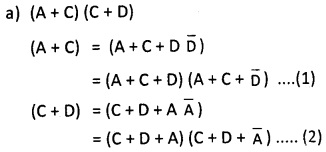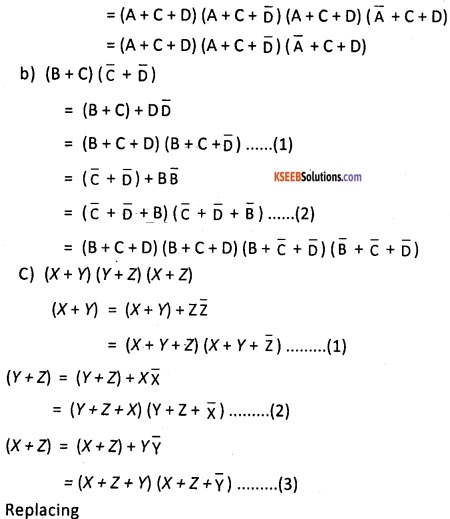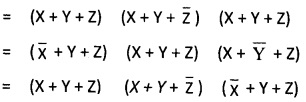Question 10.
Convert the following expressions to canonical Sum-of-Product form: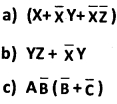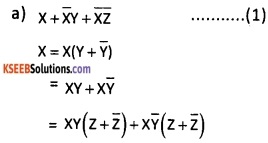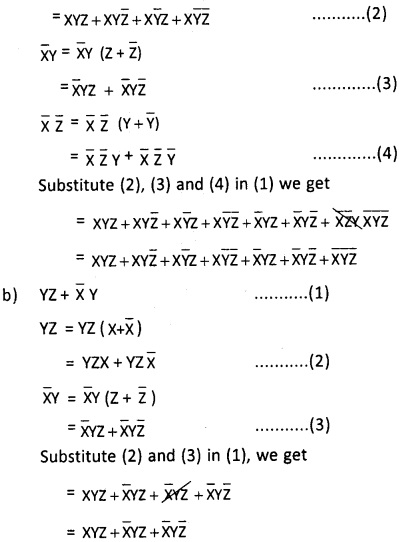Question 11.
Draw Karnaugh maps for the following expressions: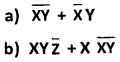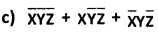Karnaugh maps for $$\bar{x} \bar{y}+\bar{x} y$$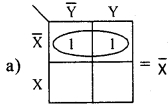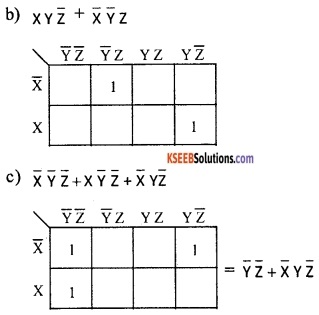Question 12.
Draw a general K-map for four variables A, B, C, and D.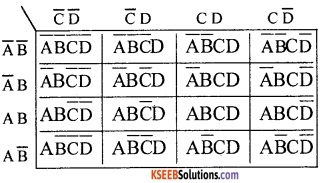Question 13.
Given the expression in four variables, draw the k-map for the function:

1. m2 + m3 + m7 + m9 + m11 + m13
2. m0 + m2 + m4 + m8 + m9 + m10 +m11+ m12 + m13

1. m2 + m3 + m7 + m9 + m11 + m13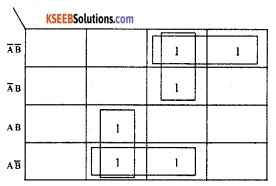2. m0 + m2 + m4 + m8 + m9 + m10 + m11 + m12 + m13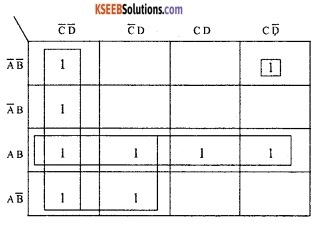Question 14.
Draw the k-map for the function in three variables given below.

1. m0 + m2 + m4 + m6 + m7
2. m1 + m2 + m3 + m5 + m7

1. m0 + m2 + m4 + m6 + m7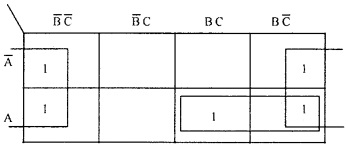2. m1 + m2 + m3 + m5 + m7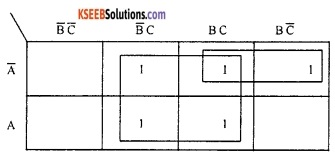Question 15.
Write SOP expression corresponding to the function F in the following truth table and draw the logic diagram (use OR and AND gates)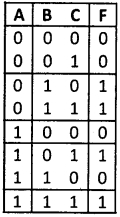SOP Expression.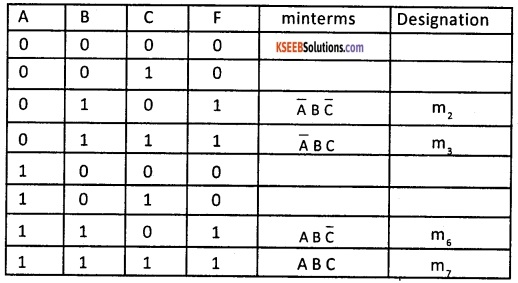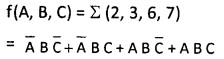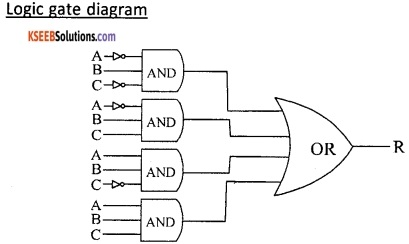### 2nd PUC Computer Science Boolean Algebra Three Marks Questions and Answers

Question 1.
State and prove any three theorems of boolean algebra.
1. Idempotance law:
This law states that when a variable is combined with itself using OR or AND operator, the output is the same variable.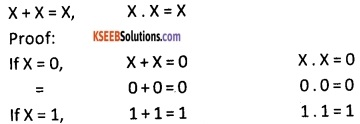2. Involution Law:
The complement of a variable is complemented again then we get the same variable.
X” = X
If X =0, then O’ = 1′ = 0
If X=1, then 1′ = 0′ = 1.3. Complementary Law:
The variable is combined with its complement and ORed and ANDed. If ORed, it always gives True (Tautology). If ANDed, then it gives False as the result always.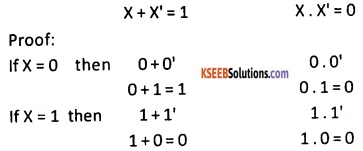Question 2.
State and prove associative law of addition and multiplication. This law states that

1. A + (B + C) = (A + B) + C
2. A. (B .C) = (A .B)

Proof: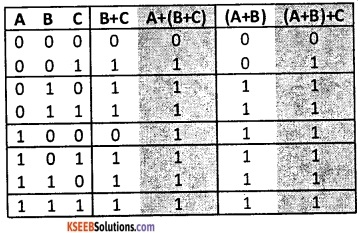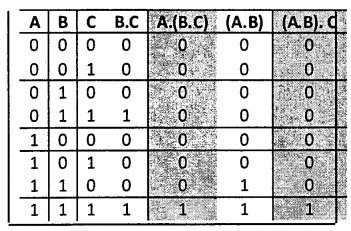Question 3.
State and prove De Morgan’s theorems by the method of perfect induction.
1. De Morgan’s First Theorem:
When the OR sum of two variables is inverted, this is the same as inverting each variable individually and then ANDing these inverted variables.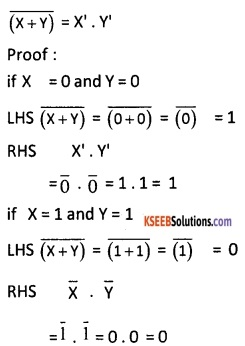2. De Morgan’s Second Theorem:
When the AND product of two variables is inverted, this is the same as inverting each variable individually and then ORing them.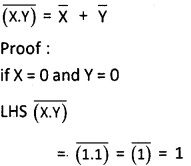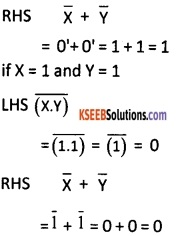Question 4.
Obtain the minterm expression for the Boolean function F = A+B’C. the minterm expression for the Boolean function F = A+B’C is
= A + B’C …….(1)
The first term A is missing two variables B and C, therefore
A = A (B + B’) = AB + AB’ = AB (C+C’) + AB’ (C+C’)
= ABC + ABC’ + AB’C + AB’C’ …….(2)
The second term B’C is missing variable A, therefore
B’C = B’C (A + A’)
= AB’C + A’B’C …….(3)
Now, Substitute (2) and (3) in (1) we get
ABC + ABC’ + AB’C + AB’C’ + AB’C + A’B’C.

Question 5.
Explain with an example how to express a Boolean function in its sum of product form.
The logical sum of two or more logical product terms is known as sum of products expression. SOP is an ORing of ofANDedvariables. The boolean expression containing all the input variables either in complemented or un complemented form in each of the product term is known as canonical SOP expression and each term is called minterm.

For example, express the product of sum from the boolean function f(x,y). For example, express the product of sum from the boolean function F(X, Y) and the truth table for which is given below: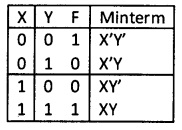Now by adding minterm for the output 1’s, we get the desired sum of product expression which is X’Y’ + XY.Question 6.
Explain with an example how to express a Boolean function in its product of sum form.
The logical product of two or more logical sum terms is known as a product of sums expression. POS is an ANDing of ORedvariables. The boolean expression containing all the input variables either in complemented or un complemented form in each of the sum term is known as a canonical POS expression and each term is called maxterm. For example, express the product of sum from the boolean function F(X, Y) and the truth table for which is given below:

 X Y F Maxterm 0 0 1 X+Y 0 1 0 X+Y’ 1 0 0 X’+Y 1 1 1 X’+Y’

Now by multiplying max terms for the output 0’s, we get the desired product of sums expression which is (X+Y1) (X’+Y).

Question 7.
Construct a truth table f r minterms and max terms for three variables and designate the terms.
The truth table for minterms for three variables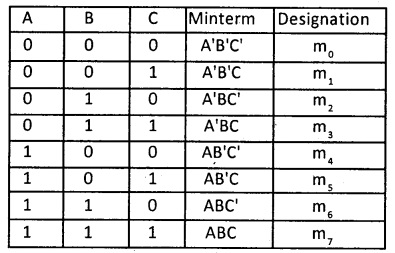The truth table for max terms for three variables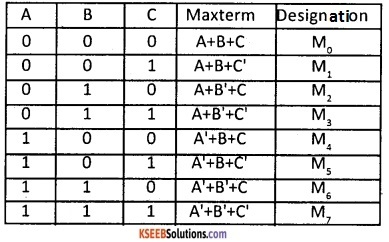Question 8.
Using basic gates, construct a logic circuit for the Boolean expression (X’+Y) (X+Z) (Y+Z)
Logic circuit for the Boolean expression ($$\bar{x}$$+Y) (X + Z) (Y + Z)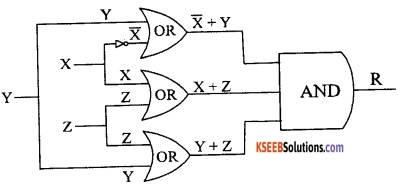Question 9.
Simplify the following Boolean expressions and draw logic circuit diagrams of the simplified expressions using only NAND gates.
Logic circuit diagram of the simplified expressions using only NAND gates.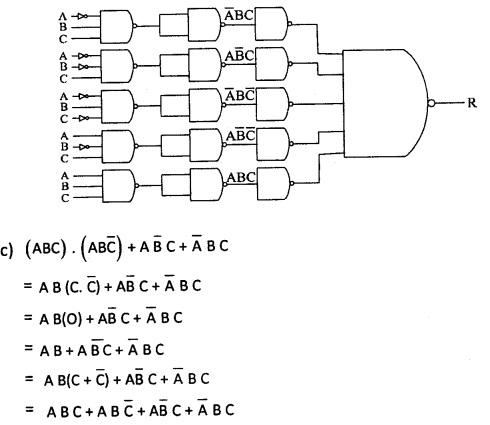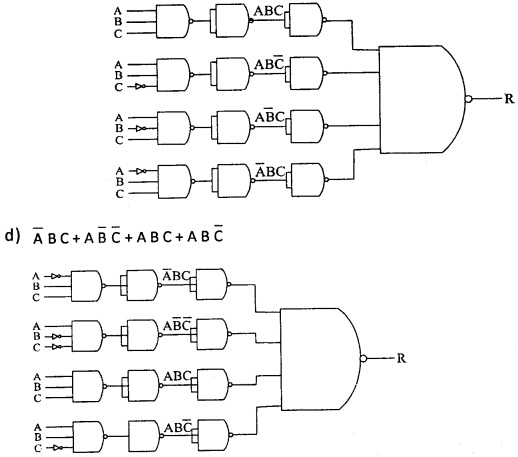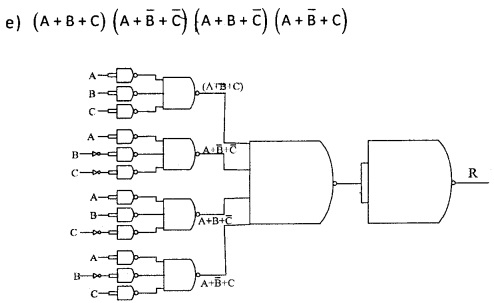Question 10.
For a four-variable map in w,x,y, and z draw the sub cubes for

1. w x$$\bar{Y}$$
2. w x
3. x y $$\bar{Z}$$ and
4. y

For a four-variable mao in w,x,y and z, the sub cubes for

1. wx$$\bar{Y}$$
2. w x
3. x y$$\bar{Z}$$ and
4. y are as follows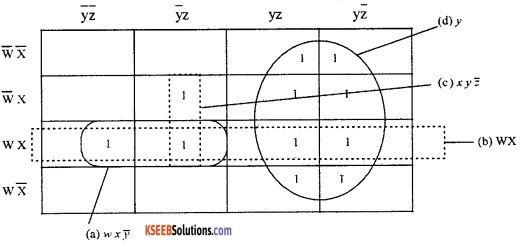Question 11.
Convert the following product-of-sums form into its corresponding sum-of-products form using truth table. F(x,y,z) = π(2,4,6,7)
POS form conversion into its SOP form using truth table is F(x, y, z) = Σ(2, 4, 6, 7)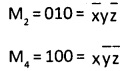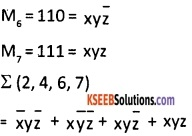Question 12.

1. Reduce the following Boolean expression to the simplest form: A. [B+C.(AB + AC)
2. Given : F(X,Y,Z) – E(l,3,7) then prove that F'(x,y,z) = π(0,2,4,5,6)

1.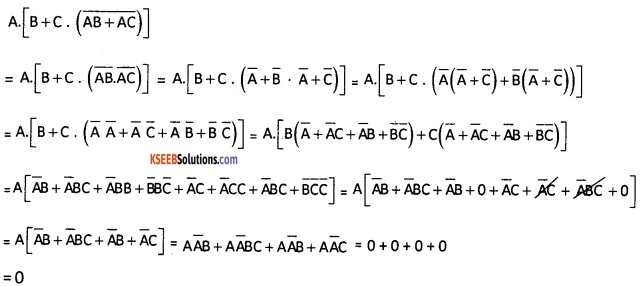2. Given F(x, y, z) = Σ(1, 3, 7)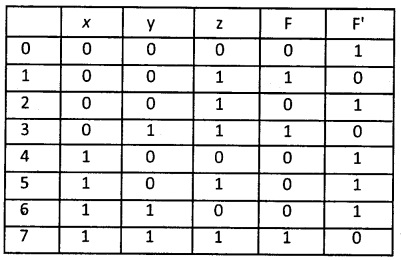From the above, the minterm list is
F(x, y, z) = Σ(1, 3, 7)
and the maxterm list is F'(x, y, z) = π(0, 2, 4, 5, 6)

### 2nd PUC Computer Science Boolean Algebra Five Marks Questions and Answers

Question 1.
Using maps, simplify the following expressions in four variables W, X, Y, and Z.

1. m1 + m3 + m5 + m6 + m7 + m9 + m11 + m13
2. m0 + m2 + m4 + m8 + m9 + m10 + m11 + m12 + m13.

1. m1 + m3 + m5 + m6 + m7 + m9 + m11 + m13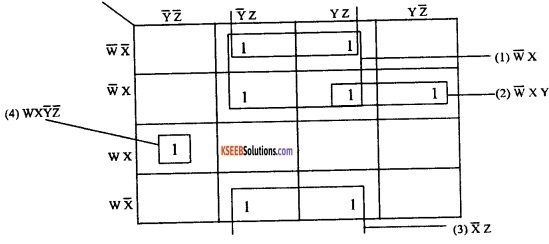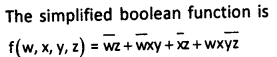2. m0 +m2 +m4 +m8 +m9 +m10 + m11 + m12 +m13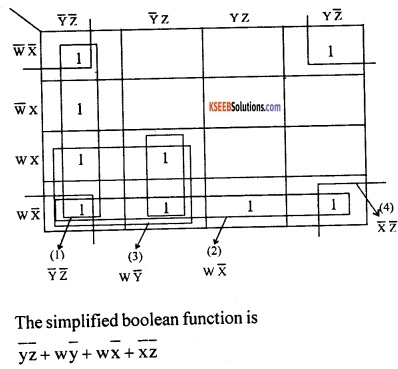Question 2.
Using maps, simplify the following expressions in four variables W, X, Y, and Z.

1. m1 + m3 + m5 + m6 + m7 + m9 + m11 + m13
2. m0 + m2 + m4 + m8 + m9 + m10 + m11 + m12 + m13.

1. m1 + m3 + m5 + m6 + m7 + m9 + m11 + m13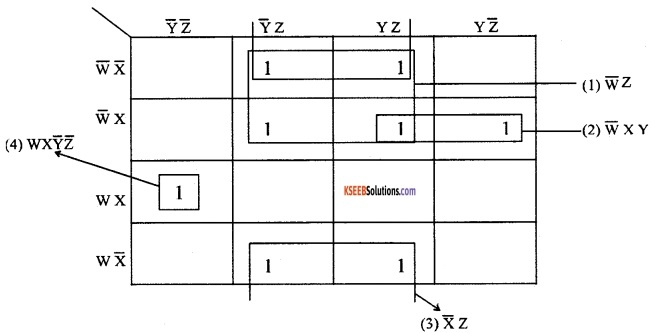2. m0 + m2 + m4 + m8 + m9 + m10 + m11 + m12 + m13.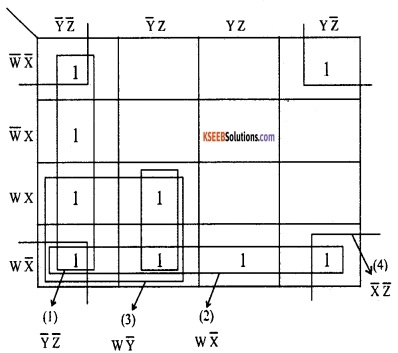Question 3.
For the Boolean function F and F’ in the truth table, find the following:
(a) List the minterms of the functions F and F’.
(b) Express F and F’ in the sum of minterms in algebraic form.
(c) Simplify the functions to an expression with a minimum number of literals.
3. a) Minterms of the functions F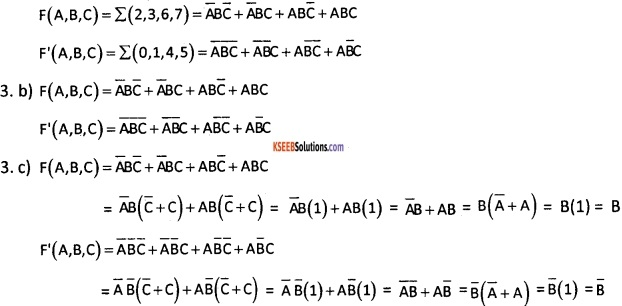Question 4.
State and prove De Morgan’s theorems algebraically.
1. De Morgan’s First Theorem:
When the OR sum of two variables is inverted, this is the same as inverting each variable individually and then ANDing these inverted variables. This is written in the born of
Boolean expression as $$\overline{x+y}=\bar{x} \cdot y$$
Proof: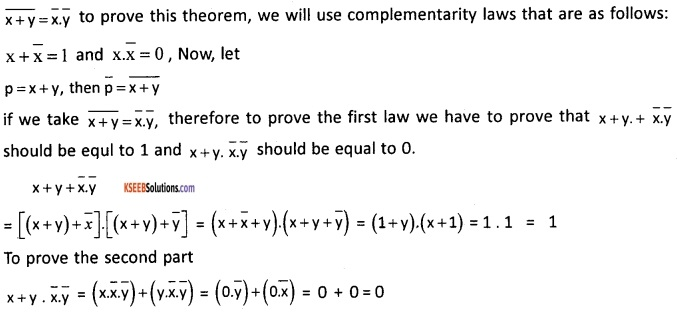2. De Morgan’s 2nd Theorem
When the AND product of two variables is inverted, this is the same as inverting each variable individually and then ORing them $$\overline{x. y}=\bar{x}+\bar{y}$$
To prove, we again use complementarity law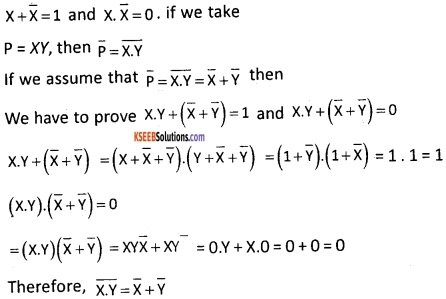Question 5.
Find the complement of F = X + YZ, then show that F.F’ = 0 and F + F’ = 1.
F = X + YZ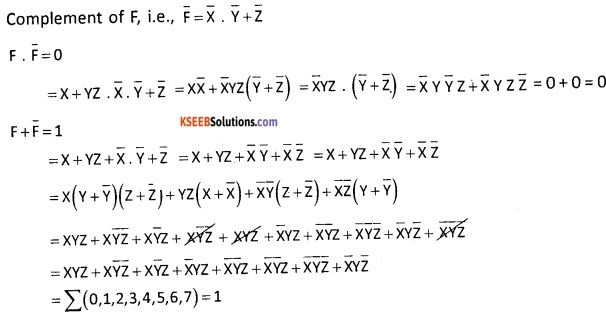Question 6.

1. State the two Absorption laws of Boolean algebra. Verify using the truth table.
2. Simplify using the laws of Boolean algebra. At each step state clearly the law used for simplification. F = x.y + x.z + x.y.z.

1. The two Absorption law of Boolean algebra are

• A +AB = A
• A (A + B) = A

Verification using truth table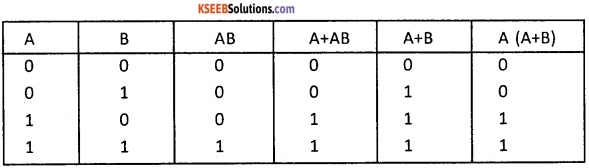2. Simplification using Boolean algebra.
F = x.y+ x.z+ x.y.z
= x(1 + y + z + yz)
= x(1) – using property of additon 1 + x = 1
= x – property of multiplication x.1 = x

Question 7.
Given the Boolean function F(x, y, z) = Σ (0, 2, 4, 5, 6). Reduce it using the Karnuagh map method.
F (x, y, z) = Σ (0, 2, 4, 5, 6)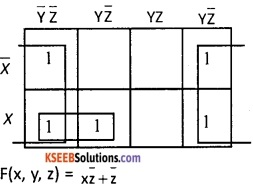Question 8.
(a) State the two complement properties of Boolean algebra. Verify using the truth tables.
(b) $$x \cdot(\overline{y z}+y z)$$
a) The two complement properties of Boolean algebra are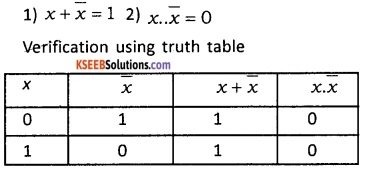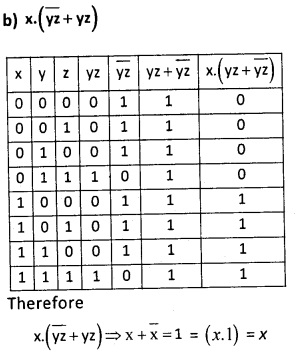Question 9.
Given the Boolean function F{A, B, C, D) = Σ(5, 6, 7, 8, 9,10,14). Use Karnaugh’s map to reduce the function F using SOP form. Write a logic gate diagram for the reduced SOP expression.